#### Transforming the robot model

Consider transforming a robot model. If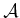is expressed by naming specific points in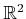, as in a boundary representation of a polygon, then each point is simply transformed from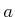to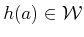. In this case, it is straightforward to transform the entire model using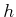. However, there is a slight complication if the robot model is expressed using primitives, such as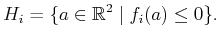(3.22)

This differs slightly from (3.2) because the robot is defined in(which is not necessarily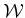), and alsois used to denote a point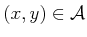. Under a transformation, the primitive is transformed as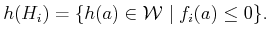(3.23)

To transform the primitive completely, however, it is better to directly name points in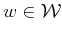, as opposed to. Using the fact that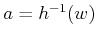, this becomes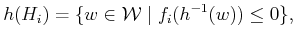(3.24)

in which the inverse ofappears in the right side because the original point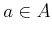needs to be recovered to evaluate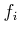. Therefore, it is important to be careful because eitheror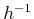may be required to transform the model. This will be observed in more specific contexts in some coming examples.

Steven M LaValle 2020-08-14